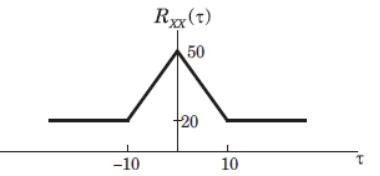Test: Random Process

# Test: Random Process

Test Description

## 10 Questions MCQ Test Analog Electronics | Test: Random Process

Test: Random Process for Electrical Engineering (EE) 2023 is part of Analog Electronics preparation. The Test: Random Process questions and answers have been prepared according to the Electrical Engineering (EE) exam syllabus.The Test: Random Process MCQs are made for Electrical Engineering (EE) 2023 Exam. Find important definitions, questions, notes, meanings, examples, exercises, MCQs and online tests for Test: Random Process below.
Solutions of Test: Random Process questions in English are available as part of our Analog Electronics for Electrical Engineering (EE) & Test: Random Process solutions in Hindi for Analog Electronics course. Download more important topics, notes, lectures and mock test series for Electrical Engineering (EE) Exam by signing up for free. Attempt Test: Random Process | 10 questions in 10 minutes | Mock test for Electrical Engineering (EE) preparation | Free important questions MCQ to study Analog Electronics for Electrical Engineering (EE) Exam | Download free PDF with solutions
 1 Crore+ students have signed up on EduRev. Have you?
Test: Random Process - Question 1

### For random process X = 6 and Rxx (t, t+t) = 36 + 25 exp(|t|). Consider following statements: (i) X(t) is first order stationary. (ii) X(t) has total average power of 36 W. (iii) X(t) is a wide sense stationary. (iv) X(t) has a periodic component. Q. Which of the following is true?

Detailed Solution for Test: Random Process - Question 1

X Constant and Rxx() is not a function of t, so X(t) is a wide sense stationary. So (i) is false & (iii) is true. Pxx = Rxx(0) 36+25 = 61. Thus (ii) is false if X(t) has a periodic component, then RXX(t) will have a periodic component with the same period. Thus (iv) is false.

Test: Random Process - Question 2

### White noise with power density No/2 = 6 microW/Hz is applied to an ideal filter of gain 1 and bandwidth W rad/s. If the output’s average noise power is 15 watts, the bandwidth W is

Detailed Solution for Test: Random Process - Question 2

Pyy = 1/2p Integral(?xx(w) |H(w)|^2 dw ) from plus infinity to minus infinity. Hence solve for W.

Test: Random Process - Question 3

### (Q.3-Q.4) The two-level semi-random binary process is defined by X(t) A or -A where (n 1)T < t < nt and the levels A and -A occur with equal probability. T is a positive constant and n = 0, ±1, ±2. The mean value E[X(t)] is

Detailed Solution for Test: Random Process - Question 3

E[X(t)] = A P(A) – (-A)P(-A) which is zero.

Test: Random Process - Question 4

The auto correlation Rxx(t1 = 0.5T, t2 = 0.7T) will be

Detailed Solution for Test: Random Process - Question 4

Here Rxx is AxA if both t1 and t2 are different and zero if they are same. Hence the answer is AxA.

Test: Random Process - Question 5

Air craft of Jet Airways at Ahmedabad airport arrive according to a Poisson process at a rate of 12 per hour. All aircraft are handled by one air traffic controller. If the controller takes a 2 – minute coffee break, what is the probability that he will miss one or more arriving aircraft?

Detailed Solution for Test: Random Process - Question 5

P (miss/or more aircraft) = 1 – P(miss 0) = 1 – P(0 arrive).

Test: Random Process - Question 6

A stationary random process X(t) is applied to the input of a system for which h(t) = u(t) t2e(-8t). If E[X(t)] = 2, the mean value of the system’s response Y(t) is

Detailed Solution for Test: Random Process - Question 6

The mean value of Y(t) is integral of h(t)dt over negative infinity to positive infinity which gives the value equal to 3/128.

Test: Random Process - Question 7

A random process is defined by X(t) + A where A is continuous random variable uniformly distributed on(0,1).

The auto correlation function and mean of the process is

Detailed Solution for Test: Random Process - Question 7

E[X(t)X(t+t)] = 1/3 and E[X(t)] = 1/2 respectively.

Test: Random Process - Question 8

(Q.8-Q.9) The auto correlation function of a stationary ergodic random process is shown below.Q.  The mean value E[X(t)] is

Detailed Solution for Test: Random Process - Question 8

Lim |t| tends to infinity, Rxx(t) = 20 = X2. hence X is sqrt(20).

Test: Random Process - Question 9

The E[X2(t)] is

Detailed Solution for Test: Random Process - Question 9

Rxx(0) = X2 = 50.

Test: Random Process - Question 10

The variance is

Detailed Solution for Test: Random Process - Question 10

Here X = 0, y = 0, Rxx(0) = 5, Ryy(0) = 10. The only value that satisfies all the given conditions is 30.

## Analog Electronics

11 videos|57 docs|108 tests
 Use Code STAYHOME200 and get INR 200 additional OFF Use Coupon Code
Information about Test: Random Process Page
In this test you can find the Exam questions for Test: Random Process solved & explained in the simplest way possible. Besides giving Questions and answers for Test: Random Process, EduRev gives you an ample number of Online tests for practice

## Analog Electronics

11 videos|57 docs|108 tests